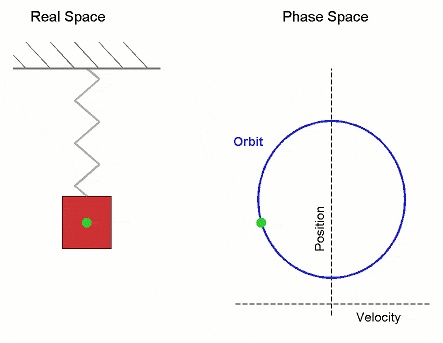# Divergence theorem in four(or more) dimension

#### enricfemi

I dont know whether it was proved or can be prove.

I dont know whether it is useful. maybe it can be used in string theory or some other things.

any comment or address will be appreciated.

#### Hurkyl

Staff Emeritus
Gold Member
What you want is (the generalized) Stoke's theorem for differential forms.

#### maze

Start with the Gauss-Green theorem. $\Omega \in \Re^d$, $u:\Re^d \rightarrow \Re$, n unit normal to $\Omega$ with components ni:

$$\int_\Omega \frac{\partial}{\partial x_i} u dx = \int_{\partial \Omega} u n_i dS$$

Now apply this theorem to each term of $\nabla \cdot F = \sum_i \frac{\partial}{\partial x_i}F_i$ to get the result on Rd.

#### enricfemi

Thanks!
How about its meaning?

#### arildno

Homework Helper
Gold Member
Dearly Missed
What is "meaning"???

It is a logical consequence of the axioms of maths we have chosen to use.

Isn't that "meaning" enough for you?

#### maze

It might have an interesting interpretation in terms of flows of probability density through a higher dimensional phase space.

For example in 2D phase space where we can visualize it, consider simple harmonic motion of a single mass on a spring:The orbit is a circle whose radius depends on how far the string stretches at the maximum. If you don't know the initial position or velocity exactly, you could consider the state of the system to be a probability distribution occupying some volume in phase space. It might be interesting to ask, how much probability is flowing into or out of a given region of phase space. Using this theorem, you could relate to the divergence of the tangent vector field inside the region of phase space to the flux through the boundary.

Now this is 2D phase space, but if you start adding in more springs and masses connected in various ways, the dimension of the phase space is going to get much bigger since you will need an axis for each position and velocity of each component.

I don't know if people who study dynamical systems actually use this, but its the first reasonable thing that I could think of.

#### Attachments

• 38.4 KB Views: 261
• 38.4 KB Views: 130
• 38.4 KB Views: 121
• 38.4 KB Views: 126
• 38.4 KB Views: 126
• 38.4 KB Views: 120
• 38.4 KB Views: 133
• 38.4 KB Views: 124
Last edited:

#### enricfemi

Special thanks to maze!

by the way, i still have a question:
for a vector field, how to define the "probability density"?
i don't think it is divergence,
but it's really exist and important. just like the density of electric field lines exhibit its strength.

### The Physics Forums Way

We Value Quality
• Topics based on mainstream science
• Proper English grammar and spelling
We Value Civility
• Positive and compassionate attitudes
• Patience while debating
We Value Productivity
• Disciplined to remain on-topic
• Recognition of own weaknesses
• Solo and co-op problem solving